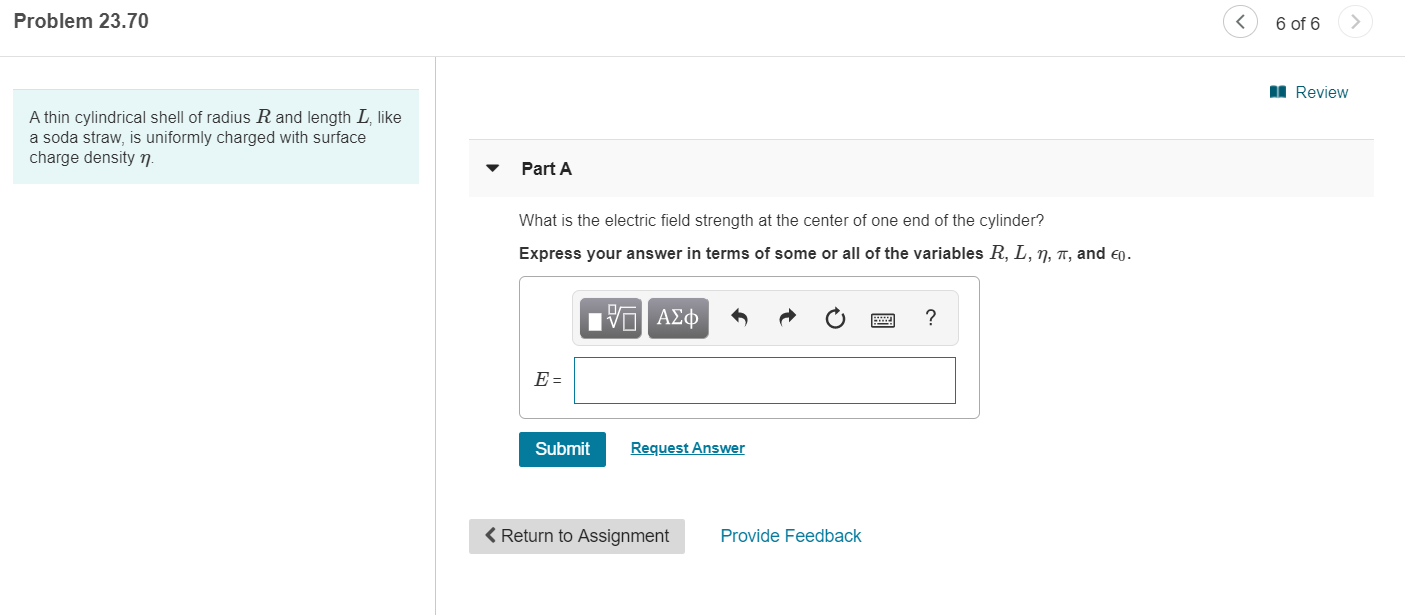# Question Solved1 AnswerProblem 23.70 6 of 6 I Review A thin cylindrical shell of radius R and length L, like a soda straw, is uniformly charged with surface charge density n. Part A What is the electric field strength at the center of one end of the cylinder? Express your answer in terms of some or all of the variables R, L, ŋ, î, and €0. Ην ΑΣφ E = Request Answer Submit < Return to Assignment Provide FeedbackTranscribed Image Text: Problem 23.70 6 of 6 I Review A thin cylindrical shell of radius R and length L, like a soda straw, is uniformly charged with surface charge density n. Part A What is the electric field strength at the center of one end of the cylinder? Express your answer in terms of some or all of the variables R, L, ŋ, î, and €0. Ην ΑΣφ E = Request Answer Submit < Return to Assignment Provide Feedback
More
Transcribed Image Text: Problem 23.70 6 of 6 I Review A thin cylindrical shell of radius R and length L, like a soda straw, is uniformly charged with surface charge density n. Part A What is the electric field strength at the center of one end of the cylinder? Express your answer in terms of some or all of the variables R, L, ŋ, î, and €0. Ην ΑΣφ E = Request Answer Submit < Return to Assignment Provide Feedback